# Generating Random Numbers in Linux Shell Scripting

1
55469There will be cases wherein you need something to be random: a random number, a random sequence of characters, a random file name, or a random password. For this purpose, you need to know the methods that will help you generate the random thing you need. Randomization will be the subject of our talk today. An interesting topic it is really, so, enjoy!!!

* * * * * *

Random Number Generation

1) Using the Random Variable

To generate a random number in a UNIX or Linux shell, the shell maintains a shell variable named RANDOM. Each time this variable is read, a random number between 0 and 32767 is generated.

To enhance the randomization process, the RANDOM variable should be initialized (seeded) to an initial value (like the process ID of the current shell, which is maintained by the variable \$\$)

Example
You need to generate 10 random numbers. How would you achieve this?

Consider the following script: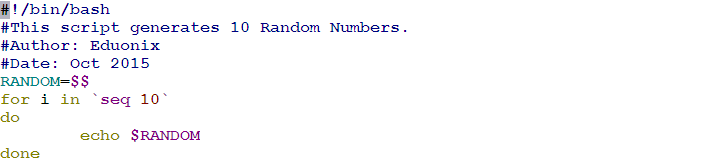Let’s execute it to see what we will get: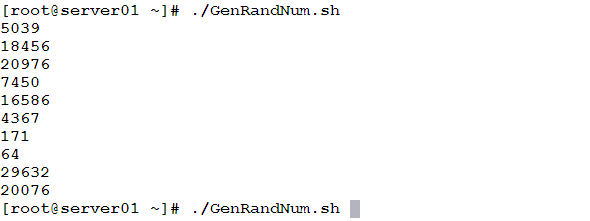Generating Random Numbers within a Range
To generate a random number in a specific range using the RANDOM variable, an intelligent trick is used. Consider we need to generate a random number that is less than an integer number Y. To do so, we use the following formula:

`R=\$((\$RANDOM%Y))`

If the range of possible numbers should include its upper limit Y, the divisor of the modulo operation should be Y+1.

```DIV=\$((Y+1))
R=\$((\$RANDOM%\$DIV))
```

Now the generated number will be between 0 and Y, inclusively.
If the generated number should be located between X and Y inclusively, where X is a non-zero integer, the formula becomes:

```DIFF=\$((Y-X+1))
R=\$((\$((\$RANDOM%\$DIFF))+X))
```

Example
The following script will accept two integers as command-line arguments and generate 5 random integers between them, inclusively.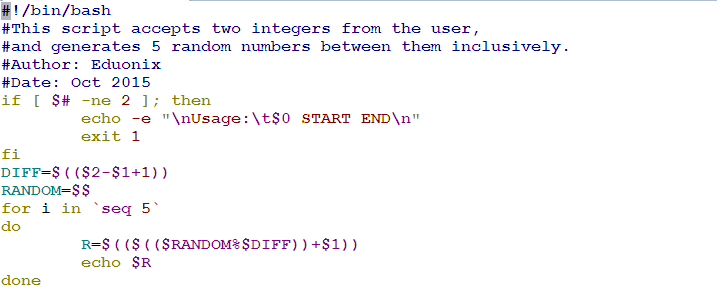Would this code achieve what we need?! Let’s see: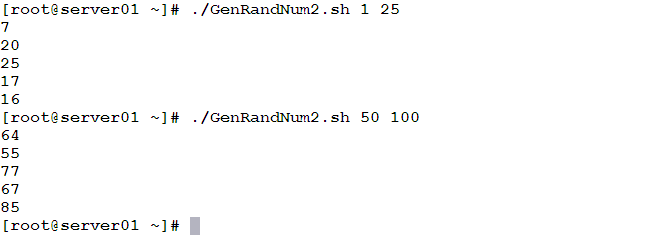It worked as expected. Now, we need to explain things: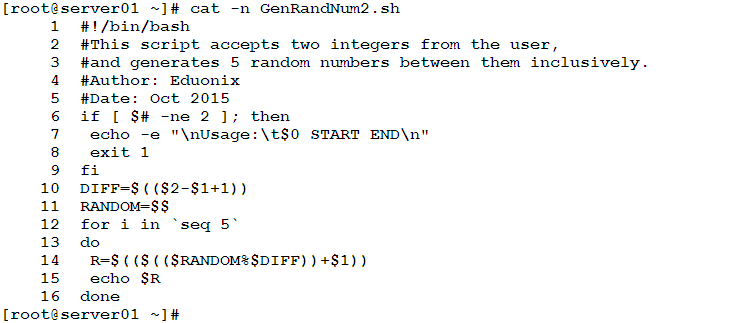• Line 6: checks the number of provided command-line arguments. If the number of arguments is not 2, a usage message is printed, and the script exits with exit code 1.
• Line 10: the lines from this until the end of the program are executed in case the user has provided the correct number of arguments (2). This line calculates the difference between the maximum and minimum range values and adds 1 to include the maximum value.
• Line 11: this line initializes the RANDOM variable to the process ID of the shell.
• Line 12: starts a for loop with a loop variable i, which loops on values from 1 to 5 (five iterations).
• Lines 13 and 16: the loop body delimiters.
• Line 14: calculates the random number from the required range according to the formula above.
• Line 15: displays the generated number.2) Using the shuf Command
Another way to generate random numbers in Linux shell is by using the shuf command. This command (according to its manual page) generates random permutations and displays them one per line.

Syntax

`shuf -i MIN-MAX -n COUNT`

Where:
MIN and MAX are the lower and upper limits of the range of numbers, respectively.
COUNT is the number of lines (random numbers) to display.

Example
The following command will generate 10 random integers from the range between 0 and 1000

`shuf -i 0-1000 -n 10`

When executed, it should give an output like the following: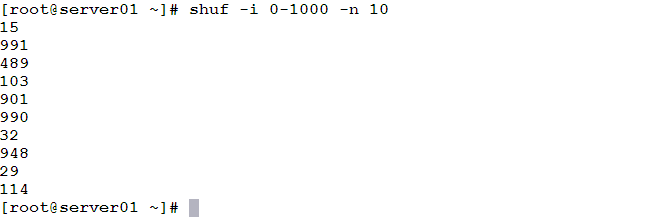3) Using /dev/random
The /dev/random special device file can be used to generate random numbers. The idea is simple: The /dev/random uses the noise collected from the device drivers and other sources to generate random data. The od (octal dump) command can extract a number of bytes and displays their decimal equivalent. That is it!!

To get one random byte (0 – 255) and display it in decimal format:

`od -An -N1 -i /dev/random`

When put inside a loop that iterates 5 times:

```[[email protected] ~]# for i in `seq 5`
> do
> od -An -N1 -i /dev/random
> done
11
248
87
52
140
[[email protected] ~]#
```

Similarly, to get 2 bytes (0 – 65535):

```[[email protected] ~]# od -An -N2 -i /dev/random
41732
[[email protected] ~]#
```

To get a larger number, increase the number of bytes to convert (the number following the –N option)

* * * * * *

Read More: 18 BEST C & C++ IDES & CODE EDITORS FOR EFFICIENT PROGRAMMING- INFOGRAPHICS ADDED!

Summary

• Generating random data may be useful in some cases.
• To generate a random number using UNIX/Linux shell, there are several methods.
• The shell maintains a shell variable RANDOM that gives a random number between 0 and 32767 each time it is read.
• Using the shuf command, we can get a list of random numbers, each printed in a separate line.
• The /dev/random special file generates random data. Together with the od command, they could be used to generate random numbers.

In the next article, we are going to learn how to generate random data and random passwords.

See you there!!

Meanwhile, If you are a beginner and want to gain in-depth knowledge about the subject, try the “Linux Shell Programming for Beginners” course. The course comes with 9.5 hours of video that covers 12 sections such as command lines and tips, customizing your shell, conditions and loops, command-line options, and much more.

1.Tim